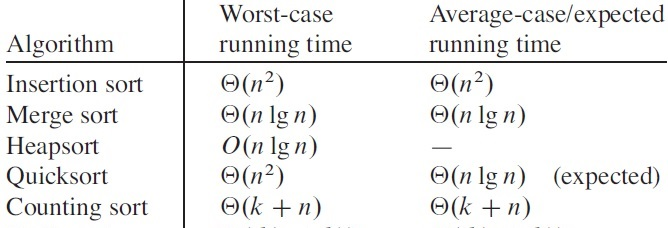CIS 5511. Programming Techniques

Sorting (2)

### 1. Quicksort

Quicksort first does a partition with a pivot value on the array to be sorted, then recursively sort the two subarrays separately.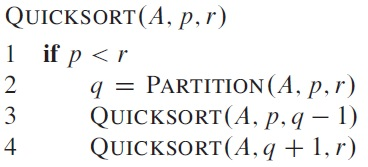The partition algorithm separates the elements of array A between index p and r that are smaller than the pivot value from the elements larger than it.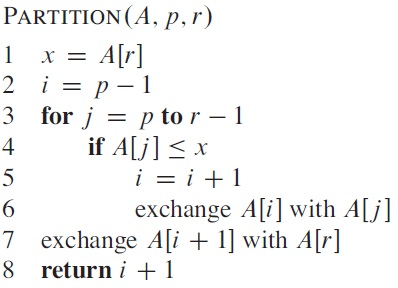Example of partition: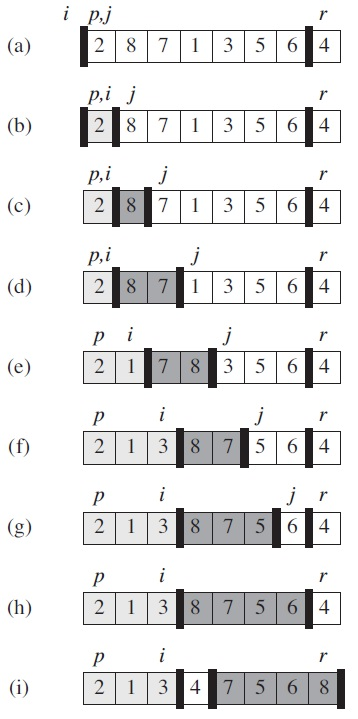Loop invariant: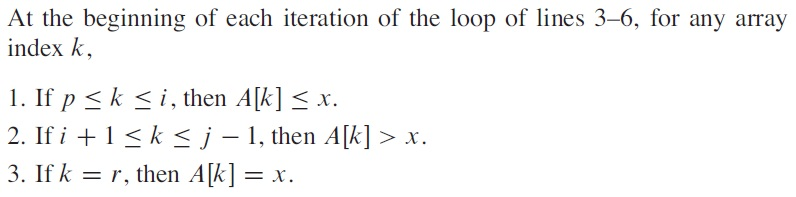Another implementation is to let two index values start at the two ends of the array, then run toward each other.

Best-case of Quicksort has T(n) ≤ 2T(n/2) + Θ(n) = O(n lg n). The result is the same even if the partition is uneven, where T(n) = T(n/a) + T(n(1 - 1/a)) + Θ(n) = O(n logan) = O(n lg n).

Worst-case of quicksort happens when the pivot values are always at the end of the array --- in that case it becomes selection sort, and the running time is T(n) = T(n-1) + Θ(n) = Θ(n2). Moreover, the worst case happens when the input is already completely sorted.

To prevent the worst-case from consistently happening, we can randomly pick pivot values from the array.

In average case, when the partitions are sometimes good and sometimes bad, the expected running time will be more similar to the best-case, that is, T(n) = O(n lg n).

### 2. Comparison sorts

All the previous sorting algorithms belong to "comparison sort", where sorting is done only by comparisons among the values to be sorted.

The major comparison sorting algorithms are summarized in the following table in their recursive forms.

 sort(first, last) linear binary with preprocessing SelectionSort select(last); sort(first, last-1); QuickSort partition(first, last); sort(first, middle-1); sort(middle+1, last); with post-processing InsertionSort sort(first, last-1); insert(last); MergeSort sort(first, middle); sort(middle+1, last); merge(first, middle, last);

Comparison sorts can be viewed in terms of decision trees. If we see each comparison as a node, then a sequence of comparisons forms a binary tree, in which each comparison (except the first one) has one parent (the preceding comparison) and two children (possible consequences). The execution of an algorithm corresponding to a path from the root to a leaf, and each leaf corresponds to a different execution path.

For a sorting problem with n items, there are n! possible input, in terms of the relative order of the items (and the absolute value of items does not matter). Therefore, the decision tree corresponding to a (comparison-based) sorting algorithm must have at least n! leaves, and the worst case corresponds to the longest path from the root to a leaf. Since a binary tree of height h has no more than 2h leaves, we have n! ≤ 2h, that is, lg n! ≤ h, while the former is Θ(n lg n) (see Page 58, Equation 3.19). Therefore, h = Ω(n lg n).

This result gives us a lower bound of the comparison-based sorting problem, independent to the algorithm used to solve the problem.

As for the best case, it is easy to see that each item must be compared at least once, so the lower bound is Ω(n), which is also the lower bound of the average case.

### 3. Non-comparison sorts

If we change the definition of the sorting problem, and assume that there is more information beside the relative order of the values, then it is possible to get faster sorting algorithms.

If the key is an integer within the range of 0 to k, we can use counting sort. The basic idea is to determine the time of occurrence of a value, then directly put it into the output.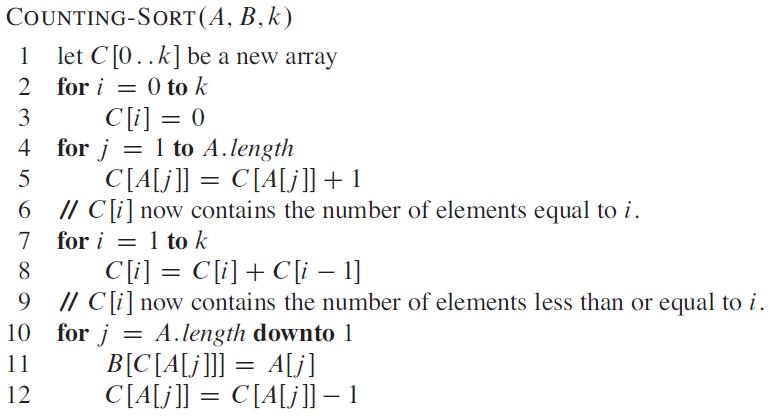Example: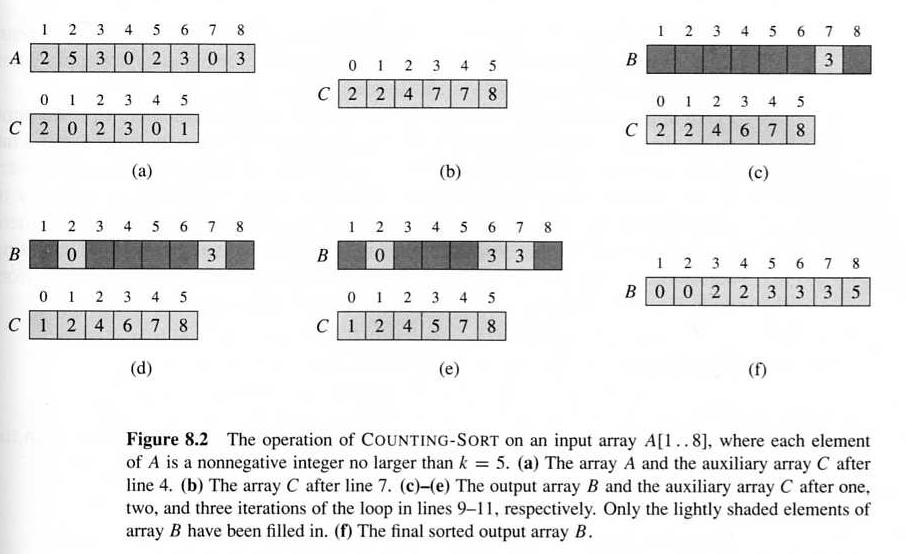The running time of the algorithm is Θ(k + n). Under the condition k = O(n), the running time is Θ(n).

There are similar sorting algorithms, and their running times are summarized in the following table: